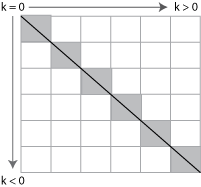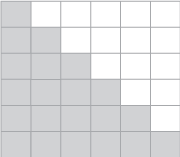# tril

Lower triangular part of matrix

## Syntax

``L = tril(A)``
``L = tril(A,k)``

## Description

example

````L = tril(A)` returns the lower triangular portion of matrix `A`.```
````L = tril(A,k)` returns the elements on and below the kth diagonal of `A`.```

## Examples

collapse all

Create a 4-by-4 matrix of ones. Extract the lower triangular portion.

`A = ones(4)`
```A = 4×4 1 1 1 1 1 1 1 1 1 1 1 1 1 1 1 1 ```
`B = tril(A)`
```B = 4×4 1 0 0 0 1 1 0 0 1 1 1 0 1 1 1 1 ```

Extract only the elements below the main diagonal.

`C = tril(A,-1)`
```C = 4×4 0 0 0 0 1 0 0 0 1 1 0 0 1 1 1 0 ```

## Input Arguments

collapse all

Input matrix.

Data Types: `single` | `double` | `int8` | `int16` | `int32` | `int64` | `uint8` | `uint16` | `uint32` | `uint64` | `logical` | `char`
Complex Number Support: Yes

Diagonals to include, specified as a scalar. `k = 0` is the main diagonal, `k > 0` is above the main diagonal, and `k < 0` is below the main diagonal.Example: `tril(A,3)`

collapse all

### Lower Triangular

The lower triangular portion of a matrix includes the main diagonal and all elements below it. The shaded blocks in this graphic depict the lower triangular portion of a 6-by-6 matrix.